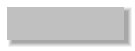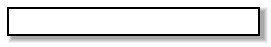The Circle And The Point

Force:
All karate styles use the circle and the point to generate force. Again, I know what you're thinking!! It is true that the traditional Japanese and Korean styles appear to be more linear in the presentation of the techniques in comparison to the Chinese styles. But make no mistake about it, the circle and the point is there. The reason it is not as obvious is that the Japanese and the Koreans practice a harder karate than do the Chinese. Let us not forget that all styles of modern day karate came from China (Shaolin suu kempo). The developers of Chinese kempo understood that force (momentum) travels in a straight line and can be economically generated by the use of the circle (rotational momentum).
In karate we train techniques which allow us to generate the greatest amount of force using the least amount of exertion. A powerful kick, punch, strike, block, or throw properly delivered is what makes karate so effective as a means of self-defense.
The following is an example of how the circle and the point work to generate force, that when applied, will travel in a straight line (see illustration
#1). First, take a tennis ball and attach a four foot string to it. Second, twirl the tennis ball over your head by holding the end of the string so that the tennis ball revolves over your head. Note, that the faster you twirl the tennis ball the more stress you are putting on the string. The reason there is more stress on the string is because the faster the tennis ball travels the more force is generated. The tension on the string is a result of the centrifugal force being applied to the tennis ball. The string is an example of the straight line in which force travels. This is best demonstrated by releasing your grip on the string which will allow the tennis ball to fly away in a perpendicular direction to the line of the string as it was when you let go of the string. The circle is the path that the tennis ball travelled while you twirled it over your head. One of the points is the exact center of the circle where your hand held the string. The other point is the exact position on the perimeter of the circle where the tennis ball was released and flew away from the perimeter of the circle. If you draw a line between the two points and then a perpendicular line forming a "T" intersection you have the direction of the force when applied.
Centrifugal force, which is not really a force, is not the force generated by this example that we employ at impact. Momentum is the force which was generated at impact. Momentum is generated when an object is set in motion. The formula is: mass of the object (weight) x speed of the object = momentum. To explain how momentum could be applied using the tennis ball, consider what would happen if you put an object in the path of the tennis ball while it is twirling. The tennis ball would strike the object establishing a point of impact. The direction of the force (momentum) applied would be on a line perpendicular to the line of the centrifugal force at the point of impact. The point of impact is but one of an infinite number of points which make up the circumference (perimeter) of the circle. To illustrate the lines of force draw a four inch circle and put a dot in the center. Next, put another dot at any point on the circle. Draw a line between the two dots. Now, draw another line at a right angle (90 degrees) to the first line connecting with the first line at the dot on the circle. You now have an illustration of how the circle and the point works in relation to the lines of force.
As I previously mentioned, (weight x speed) determines the amount of momentum. That means that either additional weight or speed must be added to generate more of that type of force. I also previously mentioned that the faster you twirl the tennis ball the more stress is put on the string. The faster you twirl the tennis ball the more force you generate. The more the tennis ball is affected by that force the harder it will strike another object. This is an important part of the physics of karate and it must not only be understood but it must be felt when executing your techniques.